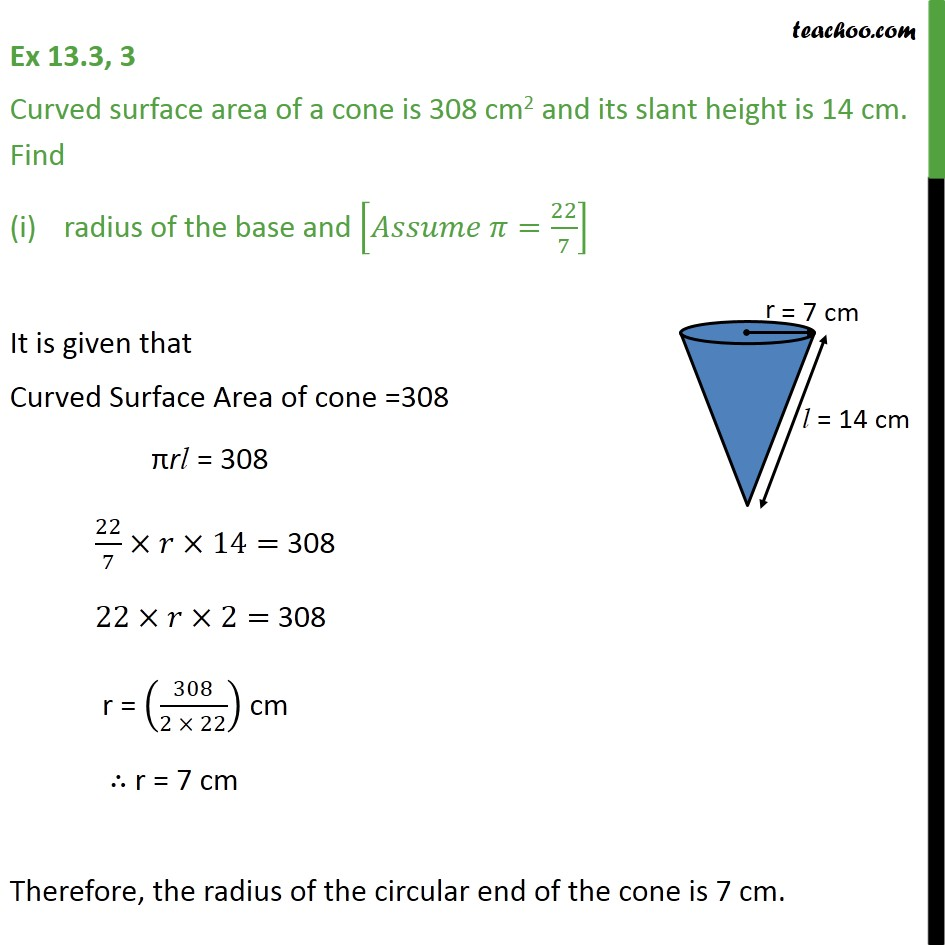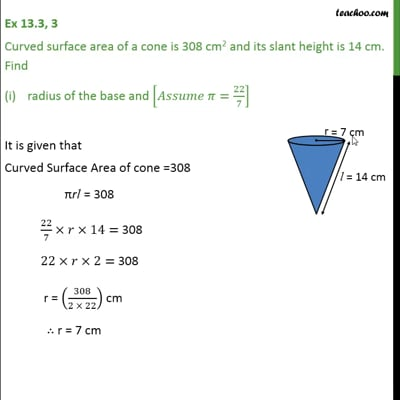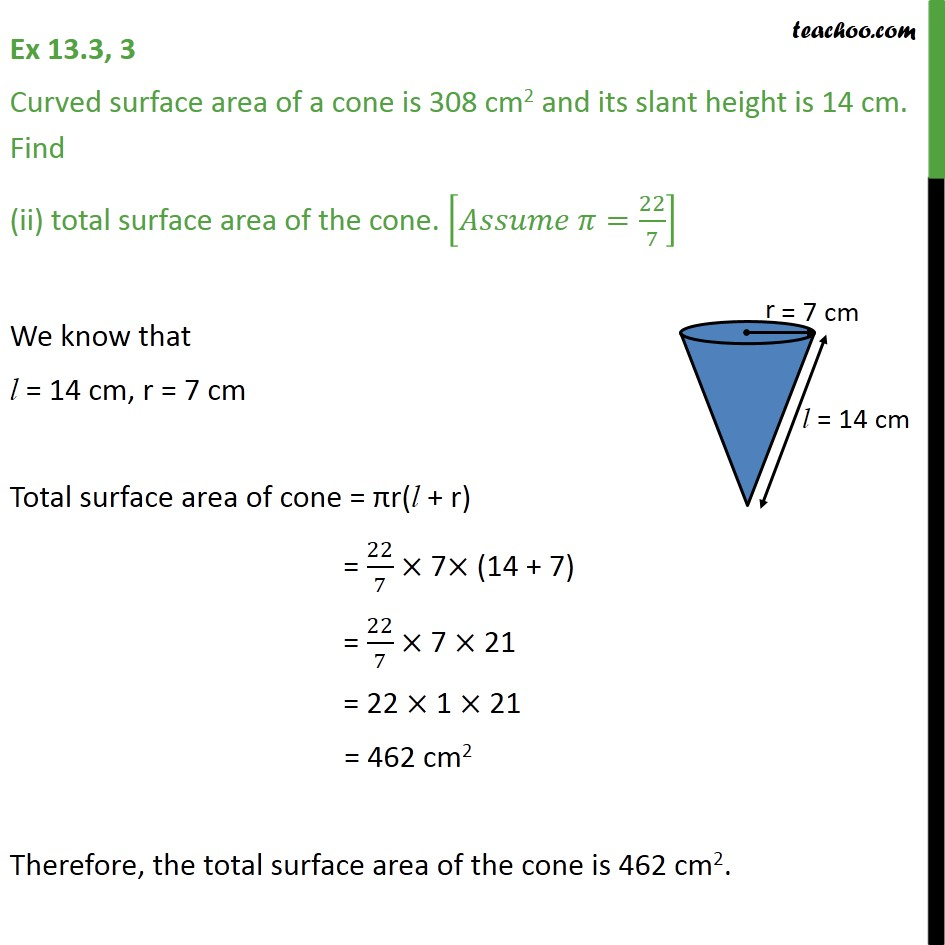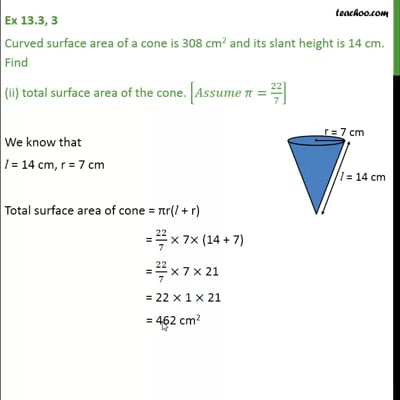Ex 13.3

Chapter 13 Class 9 Surface Areas and Volumes
Serial order wiseThis video is only available for Teachoo black usersThis video is only available for Teachoo black users

Solve all your doubts with Teachoo Black (new monthly pack available now!)

### Transcript

Ex 13.3, 3 Curved surface area of a cone is 308 cm2 and its slant height is 14 cm. Find radius of the base and [ =22/7] It is given that Curved Surface Area of cone =308 rl = 308 22/7 14= 308 22 2= 308 r = (308/(2 22)) cm r = 7 cm Therefore, the radius of the circular end of the cone is 7 cm. Ex 13.3, 3 Curved surface area of a cone is 308 cm2 and its slant height is 14 cm. Find (ii) total surface area of the cone. [ =22/7] We know that l = 14 cm, r = 7 cm Total surface area of cone = r(l + r) = 22/7 7 (14 + 7) = 22/7 7 21 = 22 1 21 = 462 cm2 Therefore, the total surface area of the cone is 462 cm2.